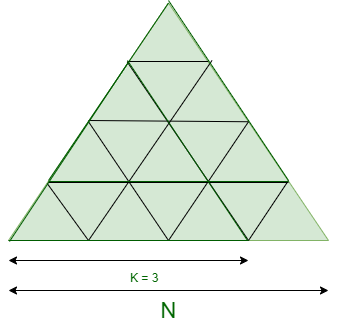Open in App
Not now

# Maximum count of Equilateral Triangles that can be formed within given Equilateral Triangle

• Difficulty Level : Expert
• Last Updated : 23 Mar, 2021

Given two integers N and K where N denotes the unit size of a bigger Equilateral Triangle, the task is to find the number of an equilateral triangle of size K that are present in the bigger triangle of side N.

Examples:

Input: N = 4, K = 3Output: 3
Explanation:
There are 3 equilateral triangles of 3 unit size which are present in the Bigger equilateral triangle of size 4 units.

Input: N = 4, K = 2
Output: 7
Explanation:
There are 7 equilateral triangles of 2 unit size which are present in the Bigger equilateral triangle of size 4 units.

Naive Approach: The idea is to iterate over all possible sizes of the bigger equilateral triangle for checking the number of triangles with the required size K and print the total count of triangles.

Time Complexity: O(N)
Auxiliary Space: O(1)

Efficient Approach: To optimize the above approach, observe the following points:

• The number of triangles with a peak in the upward direction of size K present in size N equals to ((N – K +1 ) * (N – K + 2))/2.
• The number of inverted triangles with a peak in the downward direction of size K present in size N equals to ((N – 2K + 1) * (N – 2K + 2))/2.

Below is the implementation of the above approach:

## C++

 `// C++ program for the above approach``#include ``using` `namespace` `std;` `// Function to find the number of``// equilateral triangle formed``// within another triangle``int` `No_of_Triangle(``int` `N, ``int` `K)``{``    ``// Check for the valid condition``    ``if` `(N < K)``        ``return` `-1;` `    ``else` `{` `        ``int` `Tri_up = 0;` `        ``// Number of triangles having``        ``// upward peak``        ``Tri_up = ((N - K + 1)``                  ``* (N - K + 2))``                 ``/ 2;` `        ``int` `Tri_down = 0;` `        ``// Number of inverted triangles``        ``Tri_down = ((N - 2 * K + 1)``                    ``* (N - 2 * K + 2))``                   ``/ 2;` `        ``// Total no. of K sized triangle``        ``return` `Tri_up + Tri_down;``    ``}``}` `// Driver Code``int` `main()``{``    ``// Given N and K``    ``int` `N = 4, K = 2;` `    ``// Function Call``    ``cout << No_of_Triangle(N, K);``    ``return` `0;``}`

## Java

 `// Java program for the above approach``import` `java.util.*;``class` `GFG{` `// Function to find the number of``// equilateral triangle formed``// within another triangle``static` `int` `No_of_Triangle(``int` `N, ``int` `K)``{``    ``// Check for the valid condition``    ``if` `(N < K)``        ``return` `-``1``;` `    ``else``    ``{``        ``int` `Tri_up = ``0``;` `        ``// Number of triangles having``        ``// upward peak``        ``Tri_up = ((N - K + ``1``) * (N - K + ``2``)) / ``2``;` `        ``int` `Tri_down = ``0``;` `        ``// Number of inverted triangles``        ``Tri_down = ((N - ``2` `* K + ``1``) *``                    ``(N - ``2` `* K + ``2``)) / ``2``;` `        ``// Total no. of K sized triangle``        ``return` `Tri_up + Tri_down;``    ``}``}` `// Driver Code``public` `static` `void` `main(String[] args)``{``    ``// Given N and K``    ``int` `N = ``4``, K = ``2``;` `    ``// Function Call``    ``System.out.print(No_of_Triangle(N, K));``}``}` `// This code is contributed by PrinciRaj1992`

## Python3

 `# Python3 program for the above approach` `# Function to find the number of``# equilateral triangle formed``# within another triangle``def` `No_of_Triangle(N, K):``  ` `    ``# Check for the valid condition``    ``if` `(N < K):``        ``return` `-``1``;` `    ``else``:``        ``Tri_up ``=` `0``;` `        ``# Number of triangles having``        ``# upward peak``        ``Tri_up ``=` `((N ``-` `K ``+` `1``) ``*``                  ``(N ``-` `K ``+` `2``)) ``/``/` `2``;` `        ``Tri_down ``=` `0``;` `        ``# Number of inverted triangles``        ``Tri_down ``=` `((N ``-` `2` `*` `K ``+` `1``) ``*``                    ``(N ``-` `2` `*` `K ``+` `2``)) ``/``/` `2``;` `        ``# Total no. of K sized triangle``        ``return` `Tri_up ``+` `Tri_down;``    ` `# Driver Code``if` `__name__ ``=``=` `'__main__'``:``    ``# Given N and K``    ``N ``=` `4``; K ``=` `2``;` `    ``# Function Call``    ``print``(No_of_Triangle(N, K));` `# This code is contributed by sapnasingh4991`

## C#

 `// C# program for the above approach``using` `System;``class` `GFG{` `// Function to find the number of``// equilateral triangle formed``// within another triangle``static` `int` `No_of_Triangle(``int` `N, ``int` `K)``{``    ``// Check for the valid condition``    ``if` `(N < K)``        ``return` `-1;` `    ``else``    ``{``        ``int` `Tri_up = 0;` `        ``// Number of triangles having``        ``// upward peak``        ``Tri_up = ((N - K + 1) * (N - K + 2)) / 2;` `        ``int` `Tri_down = 0;` `        ``// Number of inverted triangles``        ``Tri_down = ((N - 2 * K + 1) *``                    ``(N - 2 * K + 2)) / 2;` `        ``// Total no. of K sized triangle``        ``return` `Tri_up + Tri_down;``    ``}``}` `// Driver Code``public` `static` `void` `Main(String[] args)``{``    ``// Given N and K``    ``int` `N = 4, K = 2;` `    ``// Function Call``    ``Console.Write(No_of_Triangle(N, K));``}``}` `// This code is contributed by Rajput-Ji`

## Javascript

 ``

Output:

`7`

Time Complexity: O(1)
Auxiliary Space: O(1)

My Personal Notes arrow_drop_up